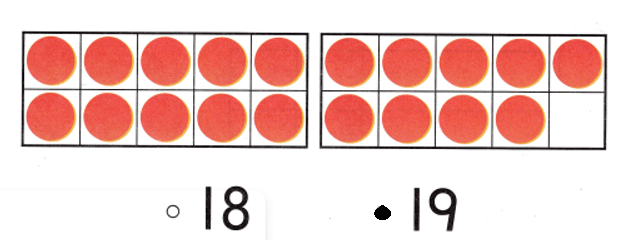# Texas Go Math Kindergarten Lesson 8.3 Answer Key Model and Count 18 and 19

Refer to our Texas Go Math Kindergarten Answer Key Pdf to score good marks in the exams. Test yourself by practicing the problems from Texas Go Math Kindergarten Lesson 8.3 Answer Key Model and Count 18 and 19.

## Texas Go Math Kindergarten Lesson 8.3 Answer Key Model and Count 18 and 19

Explore

DIRECTIONS: Use counters to show the number 18. Add more to show the number 19. Draw the counters.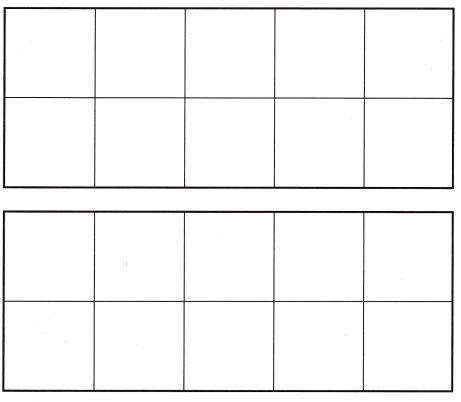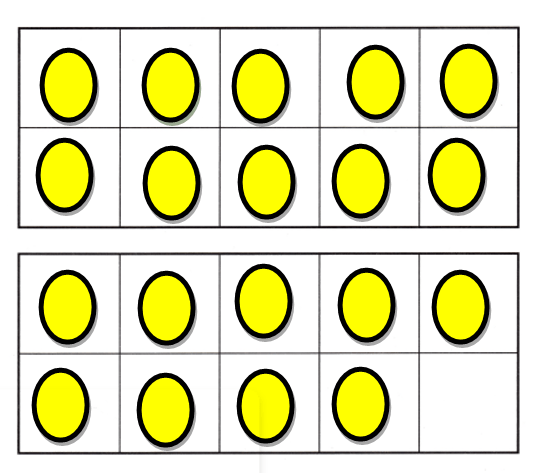Explanation:
Number of counters = 10 + 9 = 19 or Nineteen.

Share and Show

DIRECTIONS: 1. Circle 10 peaches. Tell how many more there are. 2. Place counters in the ten frames to show the number 18. Draw the counters. 3. Look at the counters you drew in the ten frames. How many ones are in the top ten frame? Write the number. How many ones are in the bottom ten frame? Write the number.

Question 1.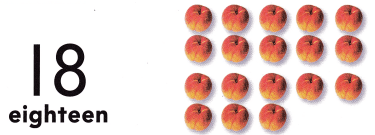Explanation:
Number of peaches = 10 + 8 = 18 or Eighteen.

Question 2.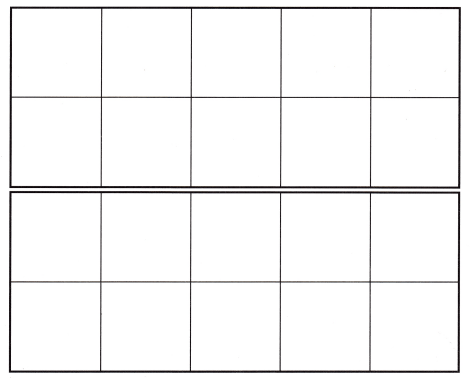Explanation:
Number of counters = 10 + 8 = Eighteen.

Question 3.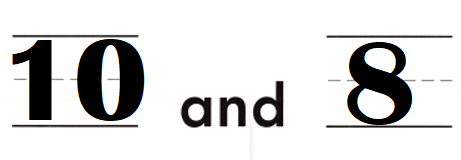Explanation:
Number of counters = 10 + 8 = Eighteen.

DIRECTIONS: 4. Circle 10 pears. 5. Place and draw counters in the ten frames to show the number 19. 6. Write the number in each ten frames.

Question 4.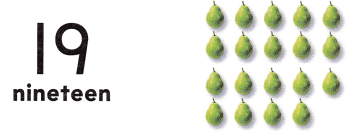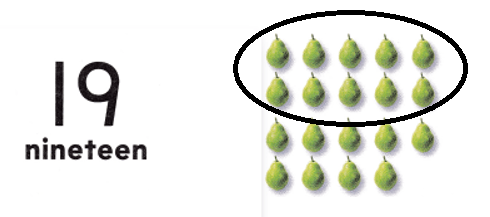Explanation:
Number of peaches = 10 + 9 = Nineteen.

Question 5.Explanation:
Number of counters = 10 + 9 = 19 or Nineteen.

Question 6.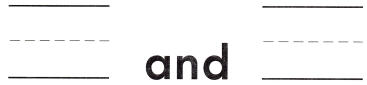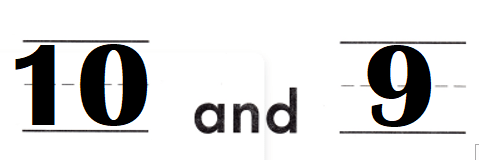Explanation:
Number of counters = 10 + 9 = 19 or Nineteen.

HOME ACTIVITY • Draw two ten frames on a sheet of paper. Have your child use small objects to model the numbers 18 and 19.Explanation:
My kid used counters to draw 18 and 19 in the frames.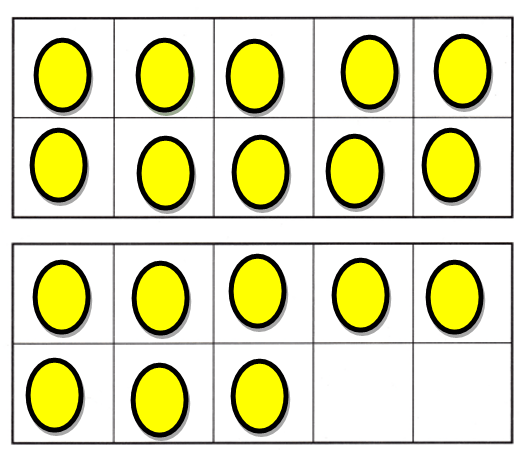Problem Solving
Question 7.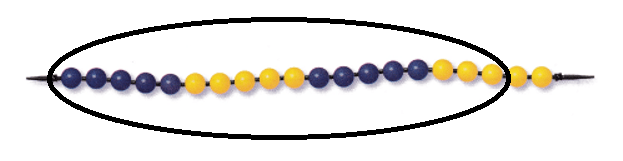Explanation:
Number of blue beads = 10.
Number of yellow beads = 8.
= 10 + 8
= 18 or Eighteen.

Question 8.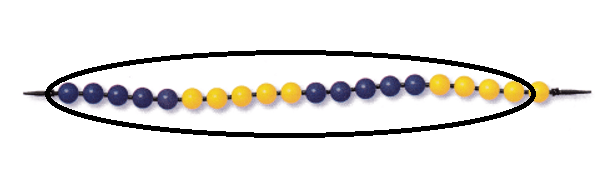Explanation:
Number of blue beads = 10.
Number of yellow beads = 9.
= 10 + 9
= 19 or Nineteen.

Question 9.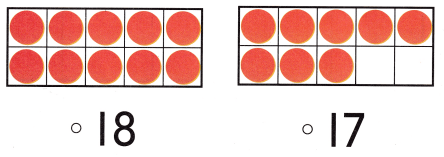Explanation:
Number of counters = 10 + 8 = 18 or Eighteen.

### Texas Go Math Kindergarten Lesson 8.3 Homework and Practice Answer Key

DIRECTIONS: 1. Draw counters to show 18. Write the number. 2. Draw counters to show 19. Write the number.

Question 1.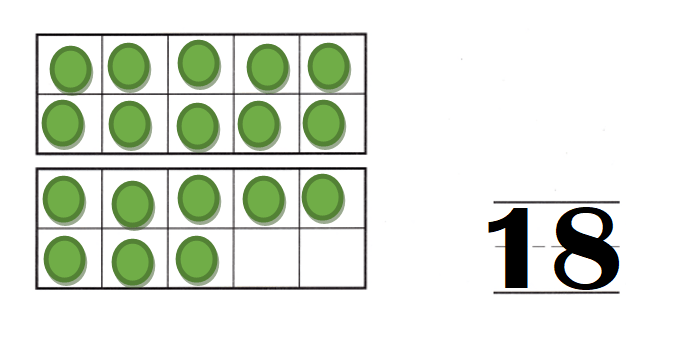Explanation:
Number of counters = 10 + 8 = 18 or Eighteen.

Question 2.Explanation:
Number of counters = 19 or Nineteen.

DIRECTIONS: Choose the correct answer. 3-5. What number does the model show?

Lesson Check
Question 3.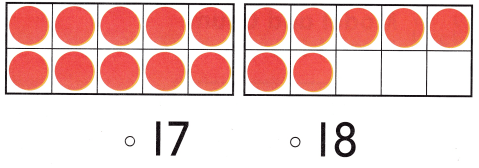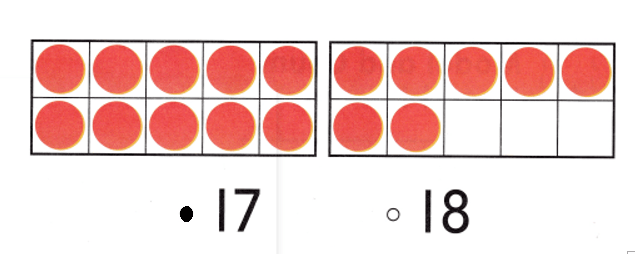Explanation:
Number of counters = 17 or Seventeen.

Question 4.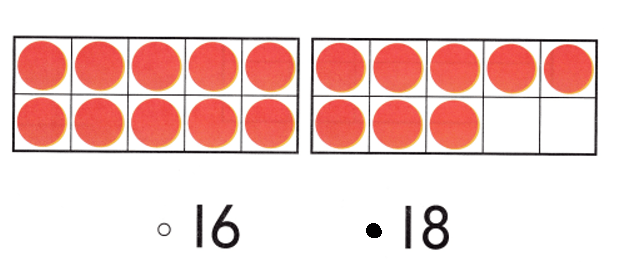Explanation:
Number of counters = 18 or Eighteen.

Question 5.